# A Preview of Calculus Lesson 1 1 What

• Slides: 18
Download presentation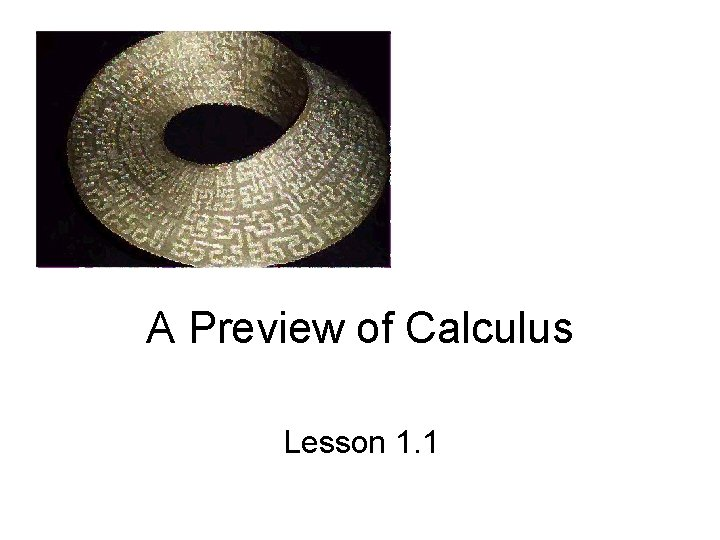A Preview of Calculus Lesson 1. 1What Is Calculus • It is the mathematics of change • It is the mathematics of – tangent lines – slopes – areas – volumes • It enables us to model real life situations • It is dynamic – In contrast to algebra/precalc which is static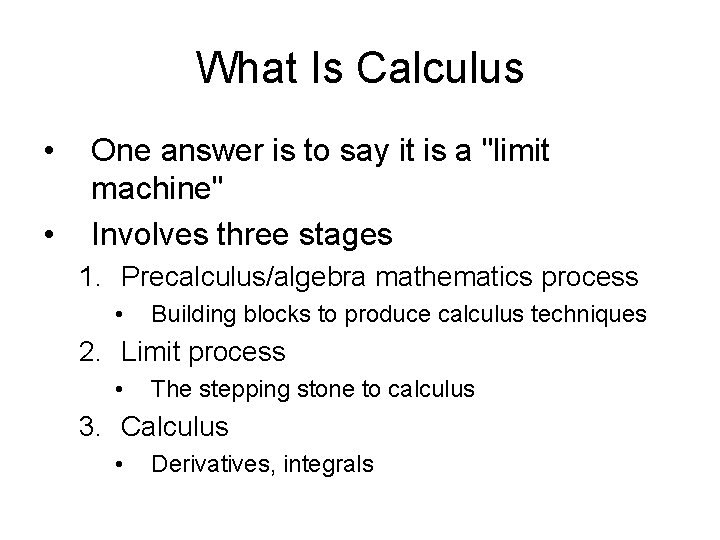What Is Calculus • • One answer is to say it is a "limit machine" Involves three stages 1. Precalculus/algebra mathematics process • Building blocks to produce calculus techniques 2. Limit process • The stepping stone to calculus 3. Calculus • Derivatives, integrals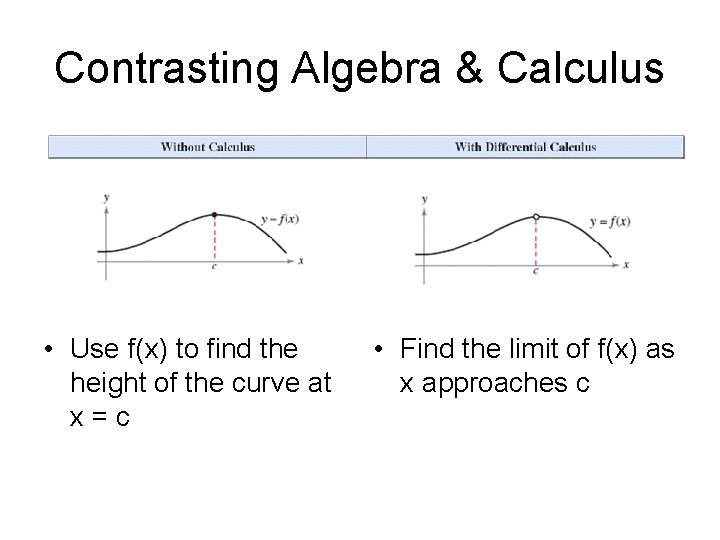Contrasting Algebra & Calculus • Use f(x) to find the height of the curve at x=c • Find the limit of f(x) as x approaches c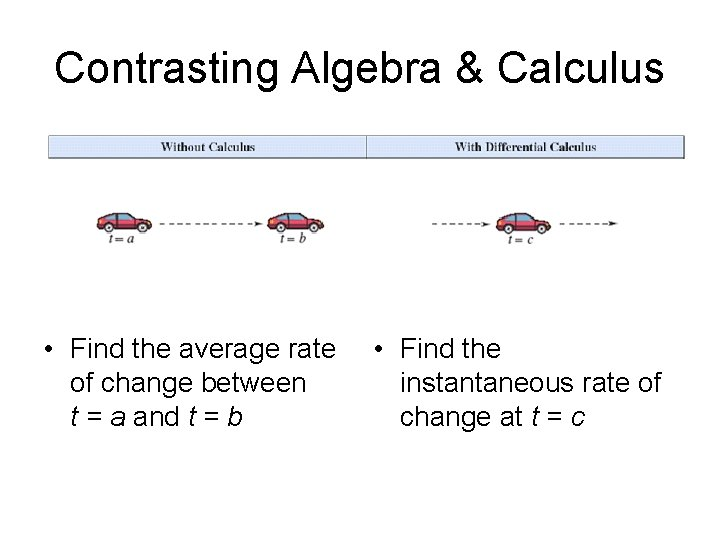Contrasting Algebra & Calculus • Find the average rate of change between t = a and t = b • Find the instantaneous rate of change at t = c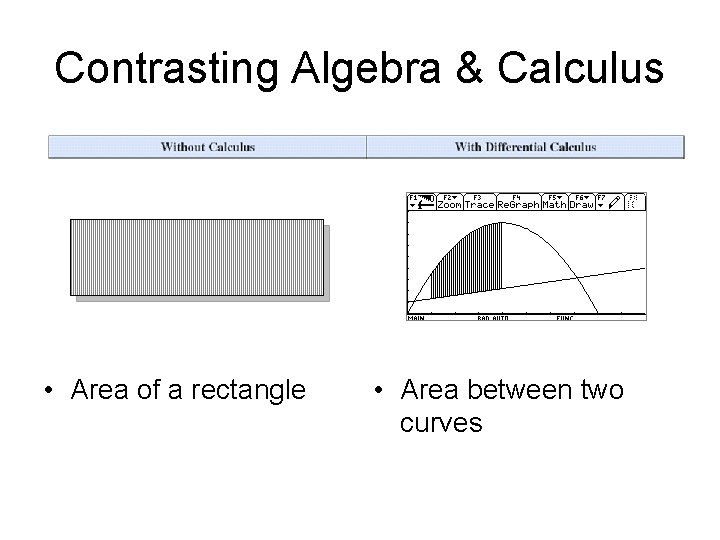Contrasting Algebra & Calculus • Area of a rectangle • Area between two curves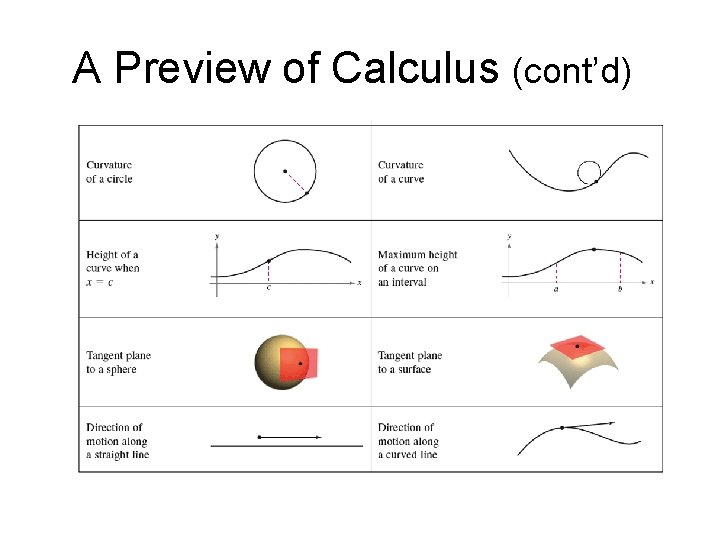A Preview of Calculus (cont’d)How do we get the area under the curve?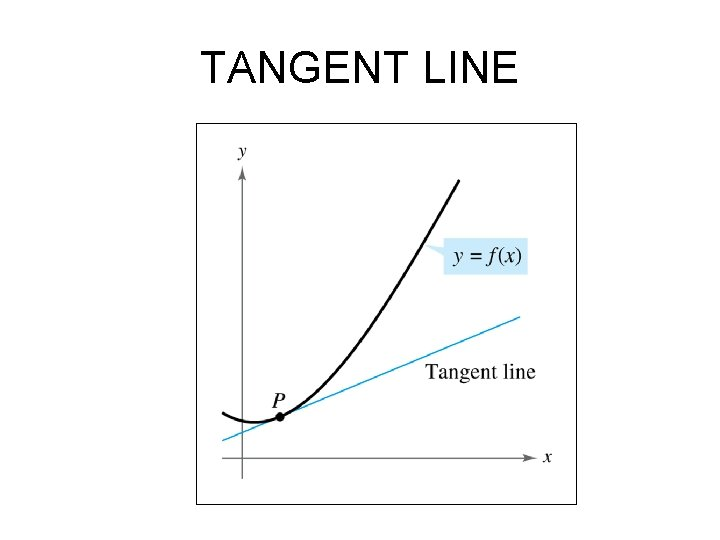TANGENT LINETangent Line Problem • Approximate slope of tangent to a line – Start with slope of secant line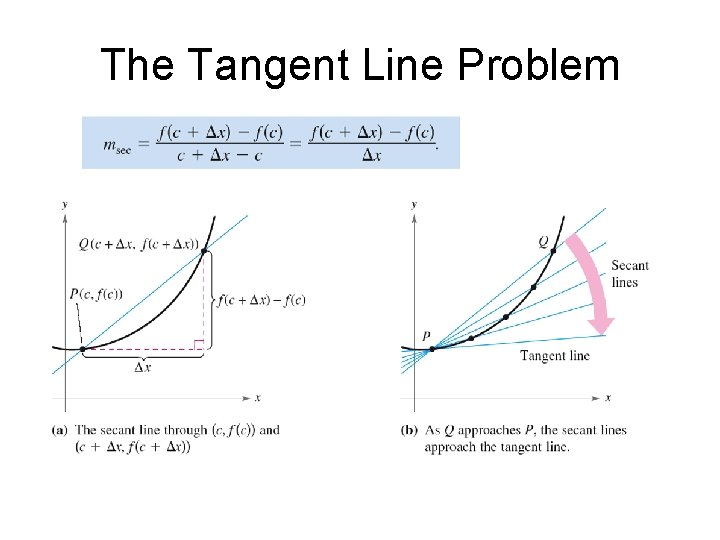The Tangent Line ProblemTangent Line Problem • Now allow the Δx to get smaller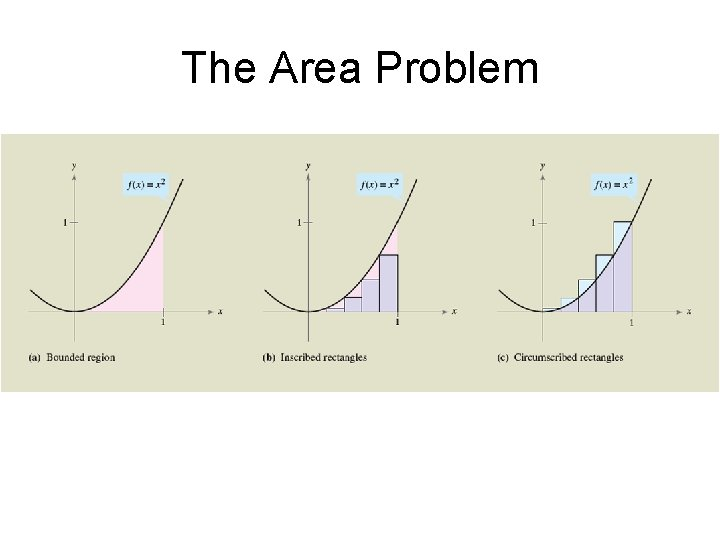The Area Problem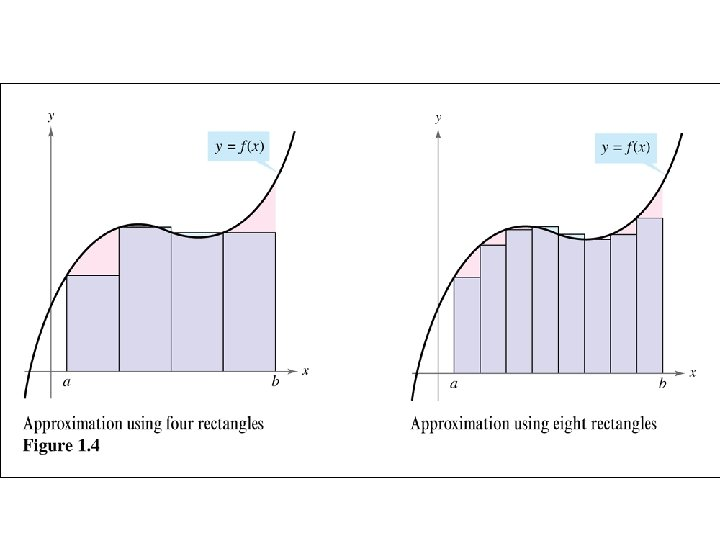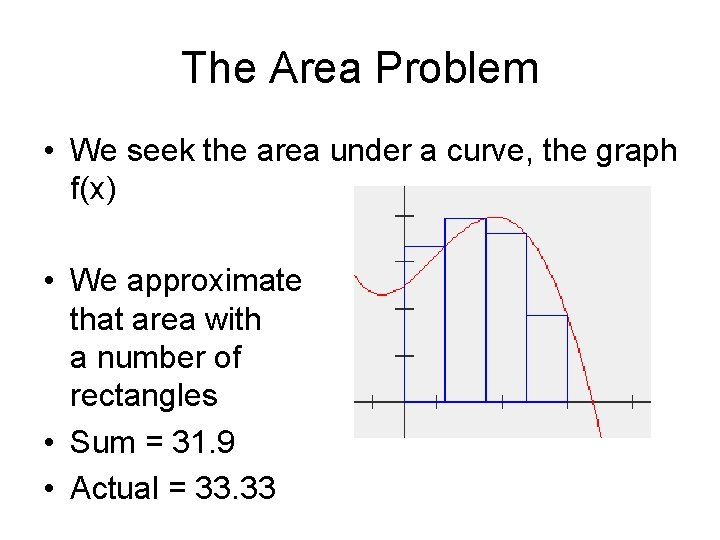The Area Problem • We seek the area under a curve, the graph f(x) • We approximate that area with a number of rectangles • Sum = 31. 9 • Actual = 33. 33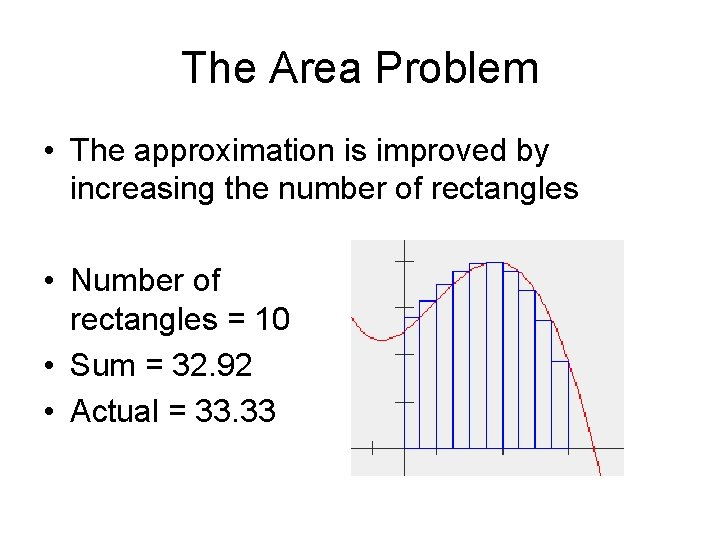The Area Problem • The approximation is improved by increasing the number of rectangles • Number of rectangles = 10 • Sum = 32. 92 • Actual = 33. 33The Area Problem • The approximation is improved by increasing the number of rectangles • Number of rectangles = 25 • Sum = 33. 19 • Actual = 33. 33• In other words!!!! As we increase the number of rectangles, we get closer and closer to the actual area of under the curve!! • Or we could say “as the limit of the number of rectangles approaches infinity”!!!! “we get closer and closer to the actual area under the curve!!!!